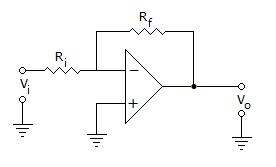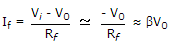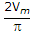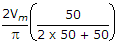# Electronics and Communication Engineering - Analog Electronics

41.

A circuit using an op-amp is shown in the given figure. It hasA. voltage series feedback B. voltage shunt feedback C. current shunt feedback D. current series feedback

Explanation:From above mathematical expression

Feedback current If is proportional to V0. So it is a voltage shunt feedback.

42.

Assertion (A): In CE amplifier the emitter is at ground potential for ac signals

Reason (R): A CE amplifier has near unity voltage gain

 A. Both A and R are correct and R is correct explanation for A B. Both A and R are correct but R is not correct explanation for A C. A is correct R is wrong D. A is wrong R is correct

Explanation:

CE amplifier has good voltage gain.

43.

An oscillator requires an amplifier

 A. with negative feedback B. with positive feedback C. with either positive or negative feedback D. none of the above

Explanation:

Positive feedback is necessary for sustained oscillations.

44.

A full wave bridge diode rectifier uses diodes having forward resistance of 50 ohms each. The load resistance is also 50 ohms. The voltage regulations is

 A. 20% B. 50% C. 100% D. 200%

Explanation:

No load output voltage =Output voltage at full load =45.

The disadvantage of direct coupled amplifiers is

 A. drift B. large size of transistor C. low voltage gain D. both (b) and (c)